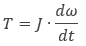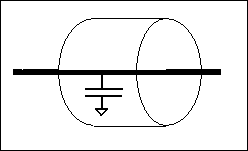OK

This component represents an inertial load and is modeled using the following characteristic equation:where

T = torque consumed by the load in Nm
ω = angular speed in rad/s
J = load inertia in kg*m2

The voltage on the component’s two pins represents the angular speed. The two pins are internally shorted.

This design allows you to chain other loads and rotary components onto the shaft while maintaining the appearance of a physical mechanical shaft. The actual loading effect is modeled using a capacitor to ground, as shown below: